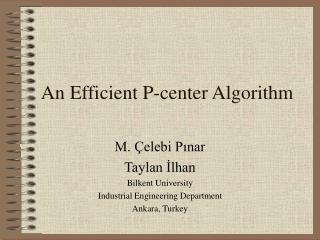DownloadDownload PresentationAn Efficient P-center Algorithm

An Efficient P-center Algorithm

Download PresentationAn Efficient P-center Algorithm

- - - - - - - - - - - - - - - - - - - - - - - - - - - E N D - - - - - - - - - - - - - - - - - - - - - - - - - - -
Presentation Transcript

1. An Efficient P-center Algorithm M. Çelebi Pınar Taylan İlhan Bilkent University Industrial Engineering Department Ankara, Turkey

2. Problem Definition & Notation • Definition:Locatingp facilities and assigning clients to them in order to minimize themaximum distance between a client and the facility to which it is assigned • Notation: • W : Set of clients • V : Set of facilities • xij : binary variable, it is 1 if client i is assigned to facility j, 0 otherwise • yj : binary variable, it is 1 if facility j is opened, 0 otherwise • wi : binary variable, it is 1 if client i is assigned to a facility, 0 otherwise • dij : distance between client i and facility j

3. Problem Formulation (PCIP)

4. Minieka(1970): solving a series of minimalsetcovering problems Daskin(2000): solving a series of maximalset covering problems Literature (Exact ones)

5. Example Formation of Set Covering

6. The proposed Algorithm • We basically solve the p-center problem by solving following feasibility problem: (IP)

7. The proposed Algorithm (cont’d.) • Our Algorithm has two parts: • PART I (LP feasibility): • Set l to minimum and u to maximum of all distance values • Threshold distance = (u-l)/2 • Define aij as follows  • Solve the LP feasibility problem(relaxing binary constraint in IP) • If the problem is feasible set u = Threshold distance else set l = Threshold distance • If (u-l) < 1 go to PART 2 else go to step 1.

8. The proposed Algorithm (cont’d.) • PART II (IP feasibility): • If the LP formulation is not feasible then set Threshold distance = u, else Threshold distance = l • Define aij as follows  • Solve the IP feasibility problem • If the problem is not feasible set new threshold distance = minimum of all distances which is greater than threshold value, and go to step 1. • Else, Stop. (Optimal Solution is found)

9. Computational Results Comparison of PCIP Formulation on CPLEX 5 with Proposed Algorithm over p-median data

10. Computational Results(Cont’d) Deviations of PCIP Formulation, its LP relaxation, and LP part of the algorithm from the optimal solution for each p-median problem instance

11. Computational Results(Cont’d) Results of the experiments with ORLIB p-median data

12. Computational Results(Cont’d) Results of the proposed algorithmwithfeasibility subproblem and maximal set coveringsubproblem

13. Future Research • Elloumi, Labbe’ and Pochet (2001) introduced a new algorithm • It is similar to the present work. Comparisons should be made.. • Extensions to more general p-center problems…

14. References • Daskin, M.S., 2000, A New Approach toSolving the Vertex P-Center Problem to Optimality: Algorithm and Computational Results, Communications of the Operations Research Society of Japan, 45:9, pp.  428-436.  • Minieka, E., 1970, The m-Center Problem , SIAM Review, 12:1. , pp. 138-139.